# Applied Mechanics and Design - Online Test

Q1. A force P is applied at a distance x from the end of the beam as shown in the figure. What would be the value of x so that the displacement at ‘A’ is equal to zero?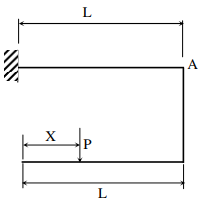Explaination / Solution:
No Explaination.

Q2. Consider a rotating disk cam and a translating roller follower with zero offset. Which one of the following pitch curves, parameterized by t, lying in the interval 0 to 2π, is associated with the maximum translation of the follower during one full rotation of the cam rotating about the center at (x, y) = (0, 0) ?
Explaination / Solution:

From all the four options the maximum amplitudes is in point ‘C’ as t = 0.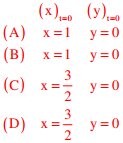Option ‘C’ has maximum net amplitude.

Q3. Consider a rotating disk cam and a translating roller follower with zero offset. Which one of the following pitch curves, parameterized by t, lying in the interval 0 to 2π, is associated with the maximum translation of the follower during one full rotation of the cam rotating about the center at (x, y) = (0, 0) ?
Explaination / Solution:

From all the four options the maximum amplitudes is in point ‘C’ as t = 0.Option ‘C’ has maximum net amplitude.

Q4. A manufacturer can produce 12000 bearings per day. The manufacturer received an order of 8000 bearings per day from a customer. The cost of holding a bearing in stock is Rs.0.20 per month. Setup cost per production run is Rs.500. Assuming 300 working days in a year, the frequency of production run should be
Explaination / Solution:
No Explaination.

Q5. A streamline and an equipotential line in a flow field
Explaination / Solution: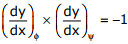Slope of equipotential Line × slope of stream function= -1
They are orthogonal to each line other.

Q6. A simply supported beam PQ is loaded by a moment of 1kN-m at the mid-span of the beam as shown in the figure. The reaction forces RP and RQ at supports P and Q respectively are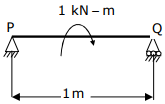Explaination / Solution: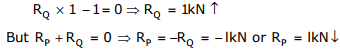Q7. A double – parallelogram mechanism is shown in the figure. Note that PQ is a single link. The mobility of the mechanism is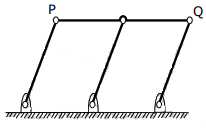Explaination / Solution:
No Explaination.

Q8. A hole is dimension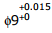mm. The corresponding shaft is of dimension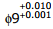mm. The resulting assembly has
Explaination / Solution:
No Explaination.

Q9. A column has a rectangular cross-section of 10mm x 20mm and a length of 1m. The slenderness ratio of the column is close to
Explaination / Solution:
No Explaination.

Q10. Match the following criteria of material failure, under biaxial stresses σ1 and σ2 and yield stress σy , with their corresponding graphic representations :
P. Maximum-normal-stress criterion             L.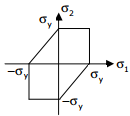Q. Maximum-distortion-energy criterion      M.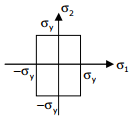R. Maximum-shear–stress criterion             N.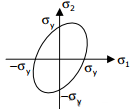Explaination / Solution:
No Explaination.